Need help? Email help@shout.education

# Reactions of Hexaaqua Ions with Ammonia Solution

This page describes and explains the reactions between complex ions of the type [M(H2O)6]n+ and ammonia solution.

It would also be useful to read two more pages: the one describing the reactions of these ions with hydroxide ions, and the one explaining ligand exchange reactions.

The present page draws information from all of these pages, and you will find it easier to understand if you spend some time reading these other pages first.

Reactions of the hexaaqua ions with ammonia solution are complicated by the fact that the ammonia can have two quite different functions. It can act as a base (in the Bronsted-Lowry sense), but it is also a possible ligand which can replace water molecules around the central metal ion. When it acts as a ligand, it is acting as a Lewis base.

We need to look at these two functions separately.

Note: If you aren't familiar with either of these terms, you should follow this link to a page about theories of acids and bases.

## Ammonia Acting as a (Bronsted-Lowry) Base

### The General Case

This is what happens when you only add small amounts of dilute ammonia solution to any of the hexaaqua ions. The ligand effect only happens with an excess of ammonia or with concentrated ammonia – and with some metals you don't even see it then.

We'll talk through what happens if you add a small amount of dilute ammonia solution to a solution containing a 2+ hexaaqua ion.

These have the formula [M(H2O)6]2+, and they are acidic. Their acidity is shown in the reaction of the hexaaqua ions with water molecules from the solution:

{\left[M(H_2O)_6\right]}^{2+} + \color{#467abf}{H_2O} \xrightleftharpoons{} {\left[M(H_2O)_5(OH)\right]}^+ + H_3O^+

They are acting as acids by donating hydrogen ions to water molecules in the solution.

Because of the confusing presence of water from two different sources (the ligands and the solution), it is easier to simplify this:

{\left[M(H_2O)_6\right]}^{2+}_{(aq)} \xrightleftharpoons{} {\left[M(H_2O)_5(OH)\right]}^+_{(aq)} + H^+_{(aq)}

#### Adding ammonia solution to this equilibrium – stage 1

There are two possible reactions.

Reaction of ammonia with the hydroxonium ions (hydrogen ions)

Ammonia will react with these to produce ammonium ions.

According to Le Chatelier's Principle, the position of equilibrium will move to the right, producing more of the new complex ion.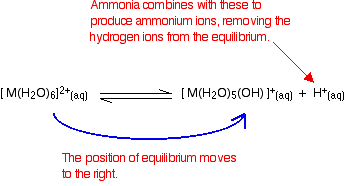Note: You really need to know about Le Chatelier's Principle, particularly with regard to the effect of changes in concentration on the position of equilibrium. Follow this link if you aren't sure.

Reaction of ammonia with the hexaaqua ion

Statistically, there is far more chance of an ammonia molecule hitting a hexaaqua metal ion than of hitting a hydrogen ion. There are far more hexaaqua ions present.

If that happens, you get exactly the same new complex ion formed as above.

{\left[M(H_2O)_6\right]}^{2+} + \color{#467abf}{NH_3} \xrightleftharpoons{} {\left[M(H_2O)_5(OH)\right]}^+ + {NH_4}^+

Notice that this is still a reversible change (unlike the corresponding change when you add hydroxide ions). Ammonia is only a weak base.

#### The second stage of the reaction

Whichever of the above reactions happens, you end up with [M(H2O)5(OH)]+ ions in solution. These are also acidic, and can lose hydrogen ions from another of the water ligands.

Taking the easier version of the equilibrium:

{\left[M(H_2O)_5(OH)\right]}^+_{(aq)} \xrightleftharpoons{} {\left[M(H_2O)_4(OH)_2\right]}_{(s)} + H^+_{(aq)}

Adding ammonia again tips the equilibrium to the right – either by reacting with the hydrogen ions, or by reacting directly with the complex on the left-hand side.

When this happens, the new complex formed no longer has a charge – this is a "neutral complex". It is insoluble in water – and so a precipitate is formed.

This precipitate is often written without including the remaining water ligands. In other words we write it as M(OH)2. A precipitate of the metal hydroxide has been formed.

#### Summarising what has happened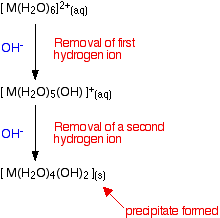You can also usefully write the complete change as an overall equilibrium reaction. This will be important for later on.

{\left[M(H_2O)_6\right]}^{2+} + \color{#467abf}{2NH_3} \xrightleftharpoons{} {\left[M(H_2O)_4(OH)_2\right]}_{(s)} + 2{NH_4}^+

If you did the same reaction with a 3+ ion, the only difference is that you would have to remove a total of 3 hydrogen ions in order to get to the neutral complex. That would give the overall equation:

{\left[M(H_2O)_6\right]}^{3+} + \color{#467abf}{3NH_3} \xrightleftharpoons{} {\left[M(H_2O)_3(OH)_3\right]}_{(s)} + 3{NH_4}^+

### Looking at the Ions of Specific Metals

Remember that we are concentrating for the moment on the ammonia acting as a base – in other words, on the formation of hydroxide precipitates when you add small amounts of ammonia solution to solutions containing hexaaqua metal ions.

The diagrams, however, will show the complete change so I don't have to repeat them later on. Ignore the cases where the precipitate dissolves in excess ammonia for the moment.

#### 2+ ions

hexaaquacobalt(II)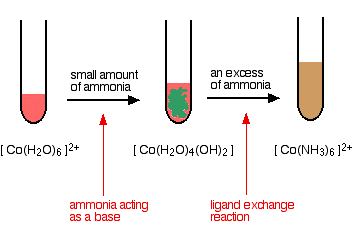Note: The final solution rapidly darkens in air to a deep red-brown. This is due to oxidation from hexaamminecobalt(II) to hexaamminecobalt(III) ions.

This oxidation is described in more detail on the page about cobalt chemistry.

hexaaquacopper(II)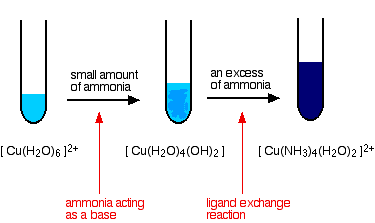hexaaquairon(II)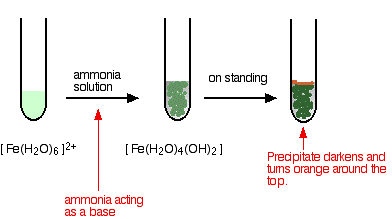Iron is very easily oxidised under alkaline conditions. Oxygen in the air oxidises the iron(II) hydroxide precipitate to iron(III) hydroxide especially around the top of the tube. The darkening of the precipitate comes from the same effect. This is NOT a ligand exchange reaction.

hexaaquamanganese(II)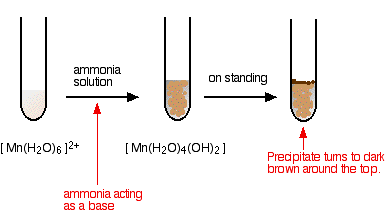I have shown the original solution as very pale pink (the palest I can produce!), but in fact it is virtually colourless. The pale brown precipitate is oxidised to a darker brown manganese(III) compound in contact with oxygen from the air. Again, this isn't a ligand exchange reaction.

Note: There is a lot of disagreement in the literature about what the darker brown compound is. It is most commonly quoted (including in a couple of research papers) as MnO(OH) or sometimes as Mn2O3,xH2O.

However, some sources quote it as a hydrated manganese(IV) oxide, MnO2,xH2O. One of the UK Exam Boards (Edexcel International) had this as a part of a question in January 2015.

hexaaquanickel(II)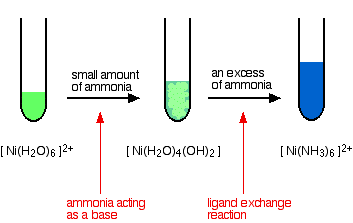hexaaquazinc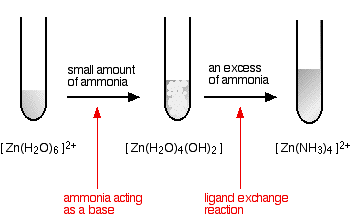You start and finish with colourless solutions, producing a white precipitate on the way.

Note: I have shown the final zinc ion as [Zn(NH3)4]2+, but I'm not certain about this. Some sources (although a minority) show it as [Zn(NH3)4(H2O)2]2+. I don't know which is right!

#### 3+ ions

hexaaquaaluminium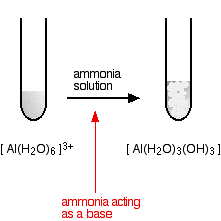Starting from a colourless solution, you get a white precipitate.

hexaaquachromium(III)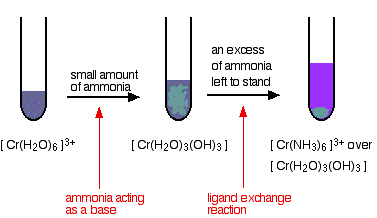hexaaquairon(III)#### Summary of the effect of adding small amounts of ammonia solution

In each case you get a precipitate of the neutral complex – the metal hydroxide. Apart from minor differences in the exact shade of colour you get, these are almost all exactly the same as the precipitates you get when you add a little sodium hydroxide solution to the solutions of the hexaaqua ions. The only real difference lies in the colour of the cobalt precipitate.

## Ammonia Acting as a Ligand

### The Ligand Exchange Reaction

In some cases, ammonia replaces water around the central metal ion to give another soluble complex. This is known as a ligand exchange reaction, and involves an equilibrium such as this one:

{\left[Cu(H_2O)_6\right]}^{2+} + 4NH_3 \xrightleftharpoons{} {\left[Cu(NH_3)_4(H_2O)_2\right]}^{2+} + 4H_2O

The formation of this new soluble complex causes the precipitate to dissolve.

The ammonia attaches to the central metal ion using the lone pair of electrons on the nitrogen atom. Because it is a lone pair donor, it is acting as a Lewis base.

### Explaining Why the Precipitate Dissolves

Almost all text books leave the argument at this point, assuming that it is obvious why the formation of the complex causes some precipitates to dissolve. It isn't! If you want to know the quite complicated reasons, read on

We'll take the copper case as typical of any of them.

There are two equilibria involved in this. The first is the one in which ammonia is acting as a base and producing the precipitate:

{\left[Cu(H_2O)_6\right]}^{2+} + 2NH_3 \xrightleftharpoons{} \left[Cu(H2O)_4(OH)_2\right] + 2{NH_4}^+

The other one is the ligand exchange reaction:

{\left[Cu(H_2O)_6\right]}^{2+} + 4NH_3 \xrightleftharpoons{} {\left[Cu(NH_3)_4(H_2O)_2\right]}^{2+} + 4H_2O

Notice that the hexaaqua ion appears in both of these. There is now an interaction between the two equilibria: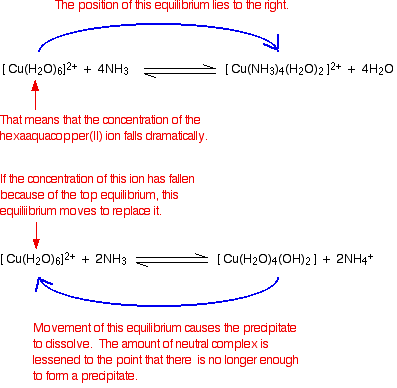Looking at it like this is helpful in explaining why some precipitates dissolve in excess ammonia while others don't. It depends on the positions of the equilibria.

To get the precipitate to dissolve, you obviously need the ligand exchange equilibrium to lie well to the right, but you need the acid-base equilibrium to be easy to pull back to the left.